# Waterloo graphics beta

Once again I’d like to welcome guest blogger Malcolm Lidierth of King’s College London. Malcolm has written several articles here, including a couple of articles on his Waterloo graphing library. Today, Malcolm discusses new features in this library, as it matures into an official beta phase.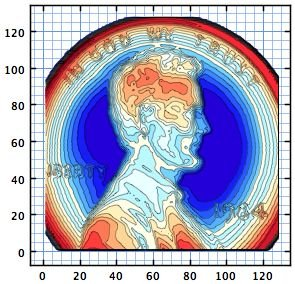Waterloo contour plot of Matlab's penny image

Last year, Yair kindly allowed me space for a couple of guest blogs on the Waterloo graphics open-source project. Waterloo has recently transitioned to a ‘beta’ release status, with several new features – many of them in response to suggestions from readers of this blog. Many thanks to all who made those.

One of the motivations in writing Waterloo was to get better, less pixellated graphs in Matlab. By using Java 2D, the core library is not tied to Matlab. Waterloo graphics can be used wherever there is access to a Java Virtual Machine: R, ScilLab etc. MathWorks obviously feel the need for better graphics too: Yair recently blogged about the next generation Matlab graphics (HG2). The Waterloo beta release provides support for mixing both Waterloo graphs and Matlab HG2 graphs in a single figure (as well as current HG1 graphics of course).

The new features in the Waterloo beta can be summarized as:

1. Introducing new plot types: contours, bars, polar and area charts
2. Mouse-selectable regions of interest
3. Support for fast-plot updates without redrawing the entire graph
4. Support for web-deployment of the graphs using SVG or Processing and ProcessingJS

Today I will concentrate on  and , illustrated with some Matlab examples; I will discuss  and  next week.

### Installation of Waterloo in Matlab

For those readers who have not yet installed Waterloo in Matlab, the process is very simple: download the latest zip file and extract it. All the sub-folders in the waterloo folder are needed but only the Waterloo_MATLAB_Library subfolder (not its subfolders) should be added to the Matlab path. Once installed, just type waterloo at the Matlab prompt in each Matlab session.

A Matlab script file that will do it all is available here (Waterloo_installer.m). The script is harmless to run if you already have Waterloo installed, but if not then it will automatically find the latest zip file on SourceForge, download and install it, and then configure the Matlab path appropriately.

### Contour plots

I ended my last guest article with an example of work-in-progress: filled contours. The beta release now fully supports these.

Recall from the previous articles that `GXFigure` creates a Waterloo-compatible Matlab figure window. gxgca() returns a reference to the container for the graph as a Matlab `GXGraph` object, much as Matlab’s built-in gca returns an axes reference.

Here is Matlab’s Lincoln penny demo in Waterloo:

```% Get some pre-defined colors colors = [kcl.waterloo.defaults.Colors.getColor(0)]; for k = 1 : 17 colors = horzcat(colors,kcl.waterloo.defaults.Colors.getColor(k)); end   f = GXFigure(); set(gcf, 'Name','Filled Contour', 'Units','normalized', 'Position',[0.3 0.3 0.4 0.4])   load penny; ax = subplot(f,1,1,1); ax.getObject().setAspectRatio(1); p2 = contourf(ax, flipud(P), 18, 'LineStyle','-', 'LineWidth',0.4); p2.getObject().setFillClipping(false); p2.getObject().setFill(colors); drawnow();```

(resulting in the contour plot above)

To transform Abe Lincoln to a logarithmic world, just double-click the graph and select the log transform. The result is shown on the right here: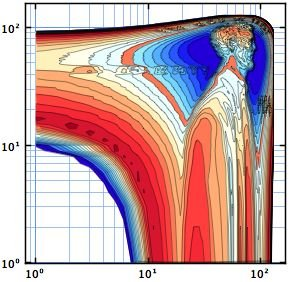Transformed Matlab penny image

All plots in Waterloo share a common data model, including contour plots. For a scatter plot, `x, y` pairs in a set represent the offsets to display a marker e.g. a circle or square that is generally of fixed size. For a contour plot, the marker is the contour line and the values for that incorporate the offsets. The `xdata` and `ydata` are added during plotting; while these will normally be zero, this makes it trivial to construct montages of contour plots simply by using non-zero values.

Plainly, this needs some extra work to support the common model: circles for a scatter plot are still painted as fixed diameter circles when the plot is rescaled or transformed but the pixel values for a contour line, bar plot etc will need to be recalculated. To achieve this:

• the data model incorporates an extra object to do the work
• such plots implement a new interface – `GJTransformUpdateInterface` – that specifies a transformUpdate() method that refreshes the pixel-coordinates. End-users will not normally need to concern themselves with this, as transformUpdate method will be called by the listeners as required.

### Categorical data

Waterloo always uses numeric data to position markers, bars etc in a plot. However, categorical data can be used to supplement those data. Here is an example using the new bar plot: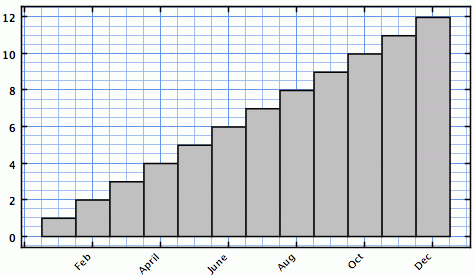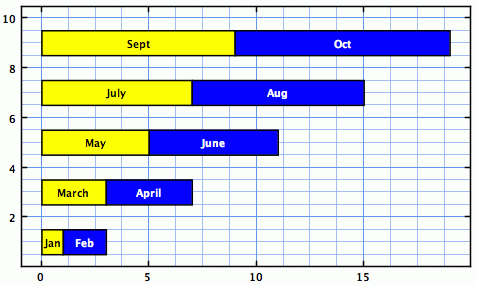Categorized Waterloo bar plots

```f = GXFigure(); set(gcf, 'Name','TestBar4', 'Units','normalized', 'Position',[0.1 0.1 0.8 0.8]); m = {'Jan', 'Feb', 'March', 'April', 'May', 'June', 'July', 'Aug', 'Sept', 'Oct', 'Nov', 'Dec'}; gr1 = subplot(f, 2, 2, 1); a = bar(gr1, 1:12, 1:12); for k = 1 : 12 a.getObject().getDataModel().getXData().setCategory(k, m{k}); end gr1.getObject().setTitleText('Label using the XData categories'); gr1.getObject().getView().autoScale();```

Support for categorical labels on the axes is supported for all plots via the common data model. For bar charts, the extra object associated with the plot also supports adding labels to the bars themselves:

```f = GXFigure(); set(gcf, 'Name','Categorized Bars', 'Units','normalized', 'Position',[0.3 0.3 0.4 0.4]); m = {'Jan', 'Feb', 'March', 'April', 'May', 'June', 'July', 'Aug', 'Sept', 'Oct', 'Nov', 'Dec'}; gr = subplot(f, 1, 1, 1); c = barh(gr, 1:10, 1:10,'stacked'); c.getObject().setFill([java.awt.Color.yellow, java.awt.Color.blue]); c.getObject().getDataModel().getExtraObject().setFontForeground([java.awt.Color.BLACK, java.awt.Color.WHITE]); for k = 1 : 12 c.getObject().getDataModel().getExtraObject().getLabels().add(k-1, m{k}); end gr.getObject().getView().autoScale();```

Note that the standard method setFill, is used to set the bar colors and as two colors are supplied the data are assumed to contain a pair of multiplexed series. This is common to all plots.

To customize the labels, we need to set a property in the extra object which is retrieved with a call to c.getObject().getDataModel().getExtraObject().

The same principles apply to pie charts:Labelled Waterloo pie chart

```f = GXFigure(); set(gcf, 'Name','TestPie1', 'Units','normalized', 'Position',[0.1 0.1 0.8 0.8]); colors = [kcl.waterloo.defaults.Colors.getColor(0)]; y = ones(1,18)*100/18; gr = subplot(f, 1, 1, 1); colors = [kcl.waterloo.defaults.Colors.getColor(1),... kcl.waterloo.defaults.Colors.getColor(17),... kcl.waterloo.defaults.Colors.getColor(2),... kcl.waterloo.defaults.Colors.getColor(16),... kcl.waterloo.defaults.Colors.getColor(3),... kcl.waterloo.defaults.Colors.getColor(15),... kcl.waterloo.defaults.Colors.getColor(4),... kcl.waterloo.defaults.Colors.getColor(14)]; c = pie(gr, [10 20 45 42 22 26 42 20], logical([0 0 1]), 'FaceColor',colors);```

### Polar charts

Polar bar and compass charts are also now supported:

```f = GXFigure(); set(gcf, 'Name','TestPie1', 'Units','normalized', 'Position',[0.1 0.1 0.8 0.8]); load sunspot.dat % Contains a 2-column vector named sunspot colors = [kcl.waterloo.defaults.Colors.getColor(0)]; for k = 1 : 17 colors = horzcat(colors,kcl.waterloo.defaults.Colors.getColor(k)); end gr1 = subplot(f, 1,2, 1); a = polarbar(gr1, sunspot(1:48,2), 'FaceColor',colors, 'EdgeWidth',0.5);   [a,b] = hist(sunspot(:,2),12); gr2 = subplot(f, 1,2, 2); b = polarbar(gr2, a, 'FaceColor',colors);   Z = eig(randn(20,20)); a = compass(gr1, real(Z), imag(Z), 'LineColor','r');```

### Area plots

Area plots are supported through a new plot class and also by having all plots implement a new Java interface. To illustrate, create two line plots:Waterloo area-fill chart

```f = GXFigure(); set(gcf, 'Name','TestAreaFill', 'Units','normalized', 'Position',[0.4 0.1 0.5 0.4]); x = 0.5 : 0.5 : 10; y = sin(x); gr1 = gxgca(); a1 = line(gr1, x, y, 'LineSpec','-ob'); b1 = line(gr1, x, y*2, 'LineSpec','-sg'); gr1.getObject().getView().autoScale();   % Filling the area between the two plots requires one extra line and a refresh call to paint the result: a1.getObject().setAreaFill(b1.getObject()); refresh();```

All the work is done in the Java code because plots now implement the `GJFillable` interface. All that is required is to call the setAreaFill() method on a class implementing `GJFillable`, specifying another `GJFillable` as input.

A new java class, `GJFill`, also implements `GJFillable` and can be used to fill an area relative to a scalar constant or an arbitrary shape. I have also written a Matlab wrapper class for this (`GXFill`, see below) but I shall use a Java-based example here.

Whether the fill is made horizontally (from the plot) or vertically (from the axes) can be selected by setting the orientation property of the `GJFill` instance. This can also be set to arbitrary, in which case we can create a custom fillable area sythesized from `java.geom` shapes: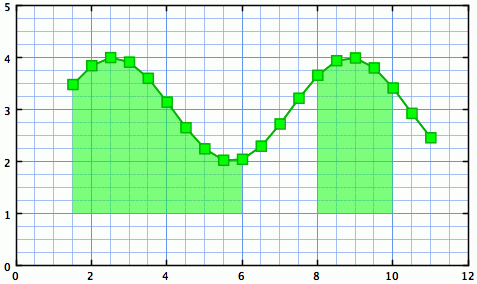Waterloo full (above) & custom (below) area fill charts

```f = GXFigure(); set(gcf, 'Name','Constant Fill', 'Units','normalized', 'Position',[0.3 0.3 0.4 0.4]); x = 0.5 : 0.5 : 10; y = sin(x); gr1 = subplot(f, 1, 1, 1); a1 = line(gxgca, x+1, y+3, 'LineSpec','-sg');   % Now create a GJFill instance, using a constant as the reference (1.5 in this case), and use this as this area's fill v = kcl.waterloo.graphics.plots2D.GJFill(a1.getObject(), 1.5); a1.getObject().setAreaFill(v);   %% Start complex % Alternately, we can use an arbitrary fill shape: v.setOrientation(javaMethod('valueOf', 'kcl.waterloo.graphics.plots2D.GJFill\$ORIENTATION', 'ARBITRARY')); a1.getObject().setAreaFill(v);   % Create a shape (which can be complex) area = java.awt.geom.Area(javaObject('java.awt.geom.Rectangle2D\$Double',1,1,5,5)); area.add(java.awt.geom.Area(javaObject('java.awt.geom.Rectangle2D\$Double',8,1,2,5)));   % Add the shape to the GJFill instance v.setArbitraryArea(java.awt.geom.Area(area)); %% End complex   % Customize the fill color v.setAreaPaint(java.awt.Color(0,1,0,0.5));   % Manually rescale and refresh the plot gr1.getObject().getView().setAxesBounds(0,0,12,5); refresh();```

To make this simpler from Matlab, a new Matlab class `GXFill` is provided. This constructs and adds a fill in a single step:

`fill = GXFill(plot_reference, value, orientation);`

where `value` is a scalar or a Java `Shape` object, and `orientation` is a string e.g. ‘horizontal’. Note that the coordinates are specified in axes units and they will rescale and be transformed as needed when the axes are changed.

### Specifying/selecting ROIs

Finally, regions of interest (ROIs) can be selected both programmatically and with the mouse. One of these can be set as the “current” ROI and that is the one that is mouse selectable: set the current ROI using shift-left mouse drag, set the region and rescale to display only that region using shift-right mouse drag.

To create an ROI that can be dragged and resized, add a `GJRoi` instance to the graph, e.g. with an existing current ROI selected:

```gr = gxgca; gr = gr.getObject().getView(); gr.add(kcl.waterloo.graphics.GJRoi.createInstance(gr, gr.getCurrentROI()));```

### Waterloo and Matlab’s Java support

Note: It appears that HG2, like HG1, creates an offscreen bitmap that is then blitted onto a Java `Canvas` within a Matlab figure. Matlab warns that the JavaFrame property will (no longer may) be discontinued in some future release, but it is my guess that this will not be the case when HG2 is released. A new set of uicontrols may indeed be included using a C-based library like wxWidgets or Qt. However, it seems unlikely that Java support will be dropped completely – too much of Matlab’s GUI uses Java (for example, the new desktop introduced in R2012b is entirely Java-based). So the Waterloo Matlab library should work, even if a switch is needed to using `JFrame`s instead of Matlab figures for output.

For the adventurous, Waterloo graphs can also be deployed using JavaFX via the SwingNode class – but that requires installation of the latest Java 8 (currently in early release status). Noting that Matlab is still (as of R2013a) using Java 6, this may indeed be a big jump (note last week’s article on upgrading Matlab to use Java 7).

Naturally, Waterloo’s graphs and classes can also be used in stand-alone Java applications, entirely outside Matlab, even on a \$30 ARM6 Raspberry Pi.

Next week, I will look at methods for animating plots (e.g. using Matlab timers) and deploying vector graphics to web pages using the in-built GUIs.Print

### 11 Responses to Waterloo graphics beta

1.Ben says:

Can you please show an example of how to annotate with an arrow?

•Ben says:

here is what I’ve tried

```f = GXFigure(); x = -5:0.1:5; gr1 = gxgca(); a1 = line(gr1, x, cos(x), 'LineSpec','-ob'); b1 = line(gr1, x, sin(x), 'LineSpec','-sg'); annotation(gcf,'arrow',[0.6 0.5],[0.2 0.4],'HeadLength',4,'LineWidth',5); gr1.getObject().getView().autoScale();```

the plot works, but not the arrow…

•Malcolm Lidierth says:

@Ben
You are creating a MATLAB annotation, not a Waterloo one, as you set “gcf” as the target. That draws a MATLAB arrow. Change gcc to gr1. Also if a HeadLength is specified you need a HeadWidth too – I’ll look at that.

Change to:

`annotation(gr1,'arrow',[0.6 0.5],[0.2 0.4],'HeadLength',4,'HeadWidth', 2, 'LineWidth',5);`

to draw a (big) arrow.

2.Dave says:

Is it possible to read images (.png) through the waterloo api? I can’t seem to find the code for it. Thanks in advance

•Dave says:

More detailed; I want to read a .png-image, add it to a MATLAB axis or FXGraph axis and have the possibility to manipulate its position and angle.

3.Peter says:

Waterloo and HG2

Waterloo (the GUI components) seems to be incompatible with the new HG2, at least in my Matlab version (2012b). For the simplest case, when I run waterloodemo I get a bunch of error messages.

Anyone have any insights?

•Malcolm Lidierth says:

@Peter
In waterloodemo comment out line 48:
set(Next.hgcontainer, ‘Opaque’, ‘off’);

In MouseMotionHandler, for all callbacks with “focus_subject” as input, explicitly cast the handle to double with
focus_subject=double(focus_subject)
on the first line of the callback.

waterloodemo should now work.

Other updates are likely to be needed for your own code as HG2 evolves.

Regards
Malcolm

4.Yousaf says:

I am making contours of temperature on a geometry of x and y axis. Could you please suggest me how could i normalize x and y axis between 0 and 1.
Thanks

5.Nino says:

Hi Yair,

First of all, thank you very much for this great website. So far, together with your 2 books (which I recommend to buy and read), it represents the best resource available about Matlab programming and software development.

I have been trying to use the Waterloo graphics on a Mac with Matlab 2015b, and I have got the following error when running the PlotGallery() test function or one of the available plot examples, either in this blog or in the Waterloo project website:

```Error using GXGraph (line 39) Java exception occurred: java.lang.NoSuchMethodError: org.jdesktop.swingx.painter.AbstractPainter.setAntialiasing(Z)V at org.jdesktop.swingx.JXLabel.initPainterSupport(JXLabel.java:287) at org.jdesktop.swingx.JXLabel.(JXLabel.java:230) at kcl.waterloo.graphics.GJAxisPanel.(GJAxisPanel.java:64) at kcl.waterloo.graphics.GJAxisPanel.(GJAxisPanel.java:147) at kcl.waterloo.graphics.GJAxisPanel.createInstance(GJAxisPanel.java:116) at kcl.waterloo.graphics.GJAbstractGraph.createAxes(GJAbstractGraph.java:469) at kcl.waterloo.graphics.GJGraphContainer.createInstance(GJGraphContainer.java:73)   Error in GXFigure/subplot (line 112) varargout{1}=GXGraph(p);   Error in gxgca (line 17) h=subplot(currentFigure, 1, 1, 1);```

I have tried to install Waterloo in both ways, manually and by using the provided script, but I keep getting the same error. In https://sourceforge.net/p/waterloo/discussion/1113980/thread/d93d580b/ I have also noticed that someone else had the same issue in the past, and after doing what Malcolm suggested in the discussion forum, the error is still there in my case.

I haven’t tried to install and use Waterloo on a Windows platform yet.

Any idea on what could cause that error and how I can fix it?

Thanks a lot,

Nino